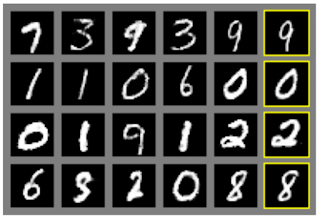2017年6月19日 星期一

GAN and Conditional GAN

GAN設計了兩個neural network: generator和discriminator，並且讓他們互相競爭來達到目的

$$\large \max\limits_{D} V(D,G) = \mathbb{E}_{x_i} \left[\log D(x) \right] + \mathbb{E}_{z} \left[ \log (1-D(G(z))) \right]$$

$$\large \min\limits_{G} \max\limits_{D} V(D,G) = \mathbb{E}_{x_i} \left[\log D(x) \right] + \mathbb{E}_{z} \left[ \log (1-D(G(z))) \right]$$

$G$ 和 $D$ 不斷競爭，最後到底誰會贏呢source: GAN papersource: GAN paper 

GAN透過競爭機制，成功得讓machine學會generative model

machine雖然能夠產生逼真的數字，可是我們卻無法指定machine產生特定的數字

Conditional GAN想要解決的問題是這樣的

Conditional GAN的架構跟GAN非常類似

Conditional GAN的數學式跟GAN的數學式非常像
$$\large \min\limits_{G} \max\limits_{D} V(D,G) = \mathbb{E}_{x_i} \left[\log D(x|y) \right] + \mathbb{E}_{z} \left[ \log (1-D(G(z|y))) \right]$$source: Conditional GAN paper 

GAN和Conditional GAN有很多的應用，等我之後看了更多的paper再來介紹給大家

Reference
 I. J. Goodfellow, J. Pouget-Abadie, M. Mirza, and B. Xu, D. Warde-Farley, S. Ozair, A. Courville, and Y. Bengio. "Generative adversarial nets." NIPS, 2014.
 M. Mirza and S. Osindero. "Conditional generative adversarial nets." Preprint arXiv:1411.1784, 2014.